# Image Segmentation Keras : Implementation of Segnet, FCN, UNet, PSPNet and other models in Keras.

Implementation of various Deep Image Segmentation models in keras.

### News : Some functionality of this repository has been integrated with https://liner.ai . Check it out!!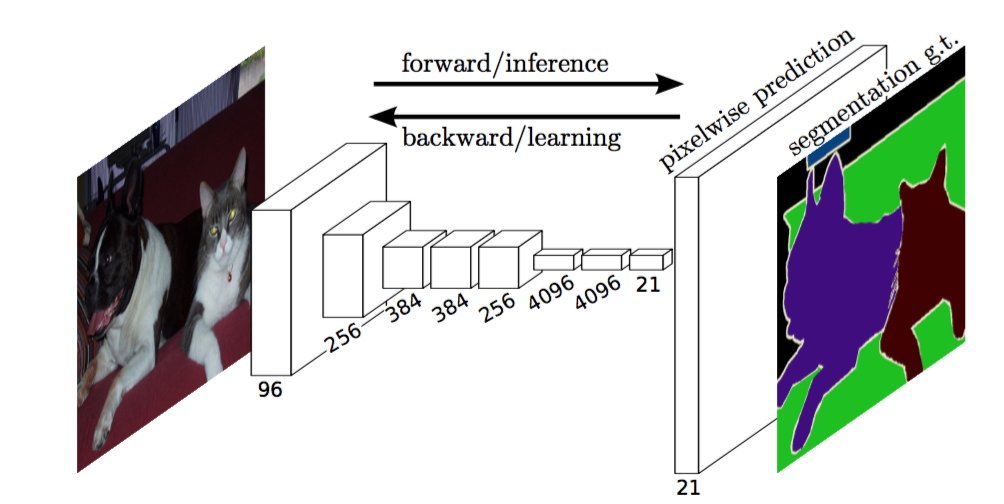Link to the full blog post with tutorial : https://divamgupta.com/image-segmentation/2019/06/06/deep-learning-semantic-segmentation-keras.html

## Training using GUI interface

You can also train segmentation models on your computer with https://liner.ai

Train Inference / Export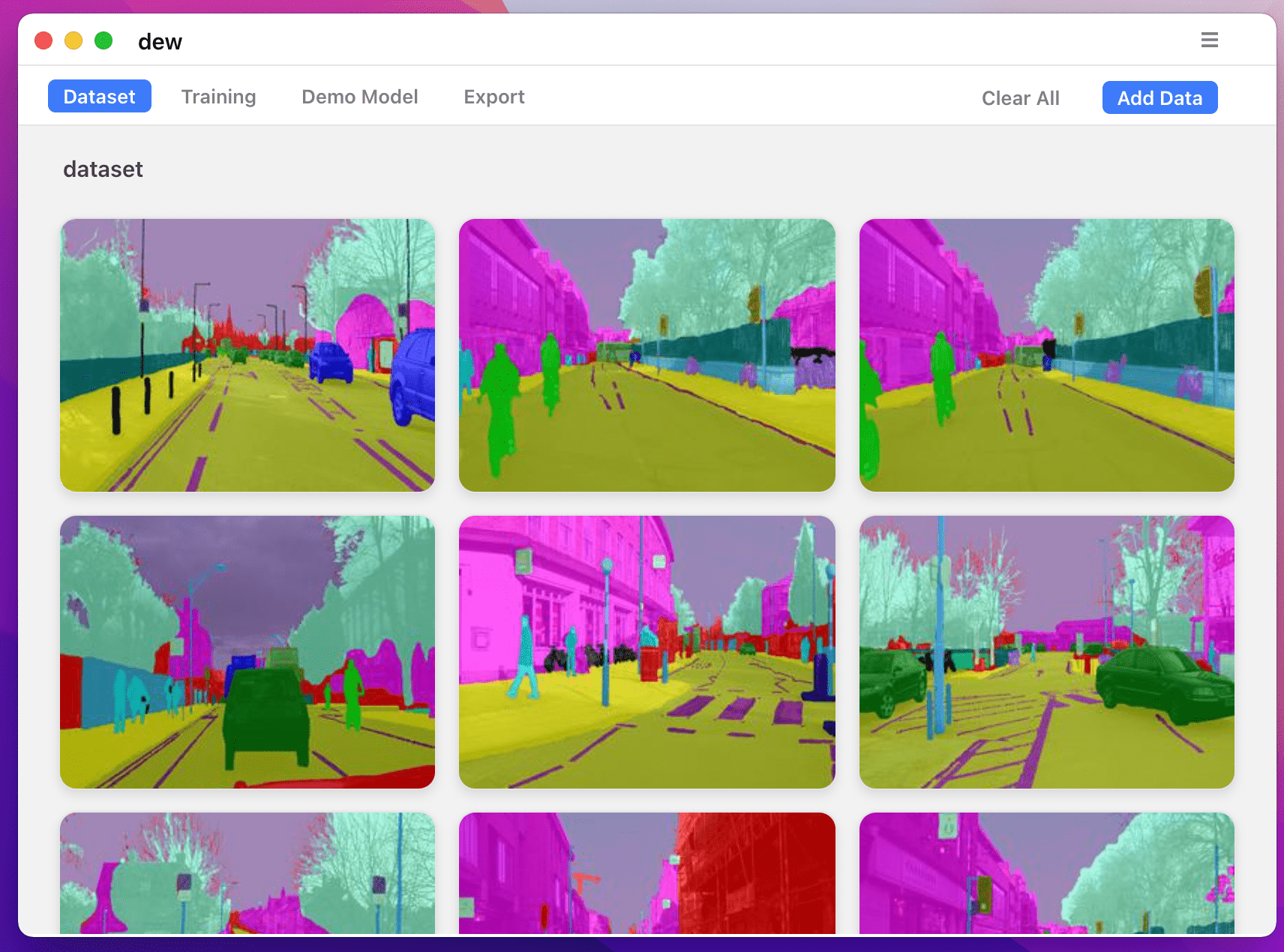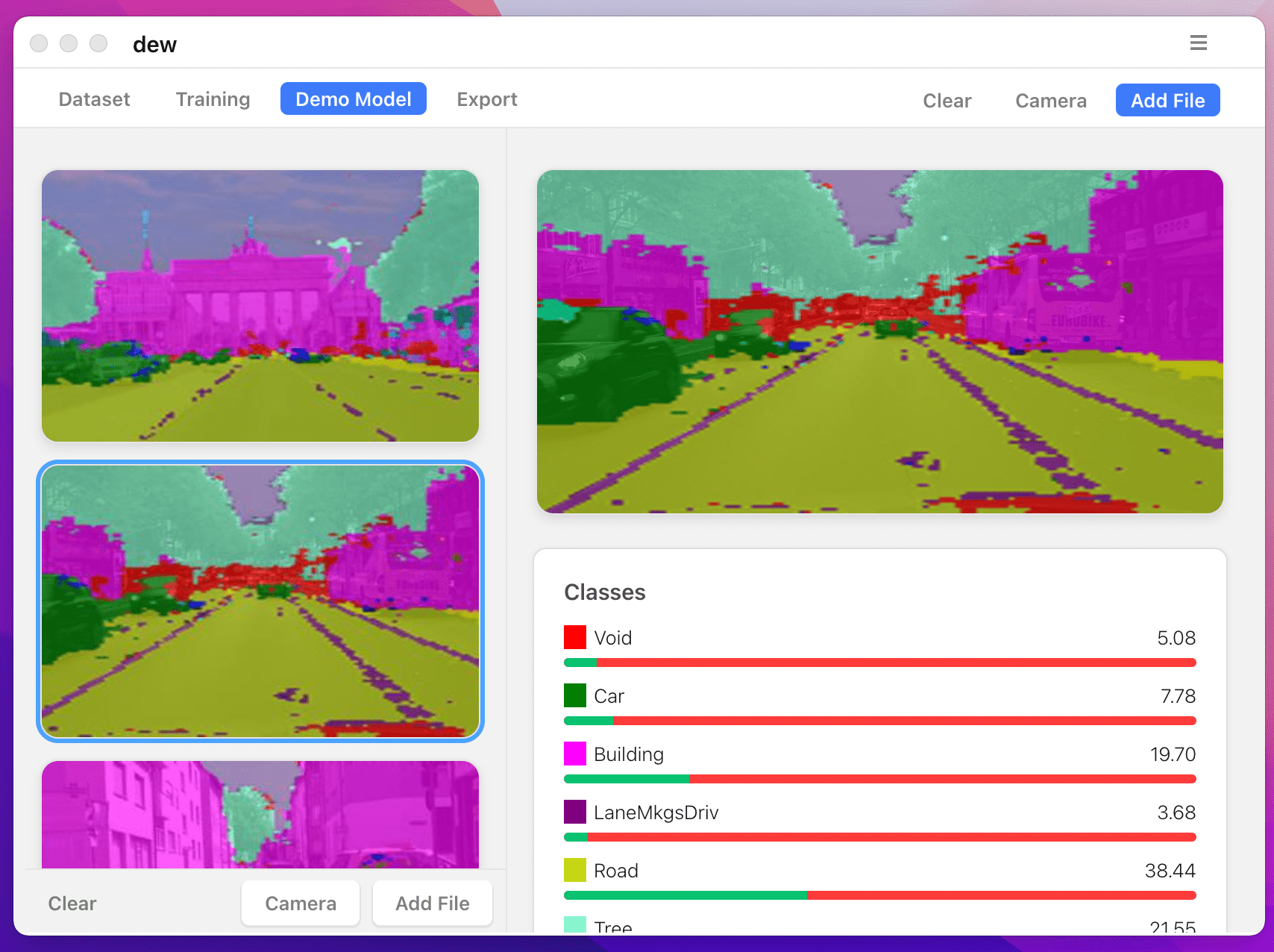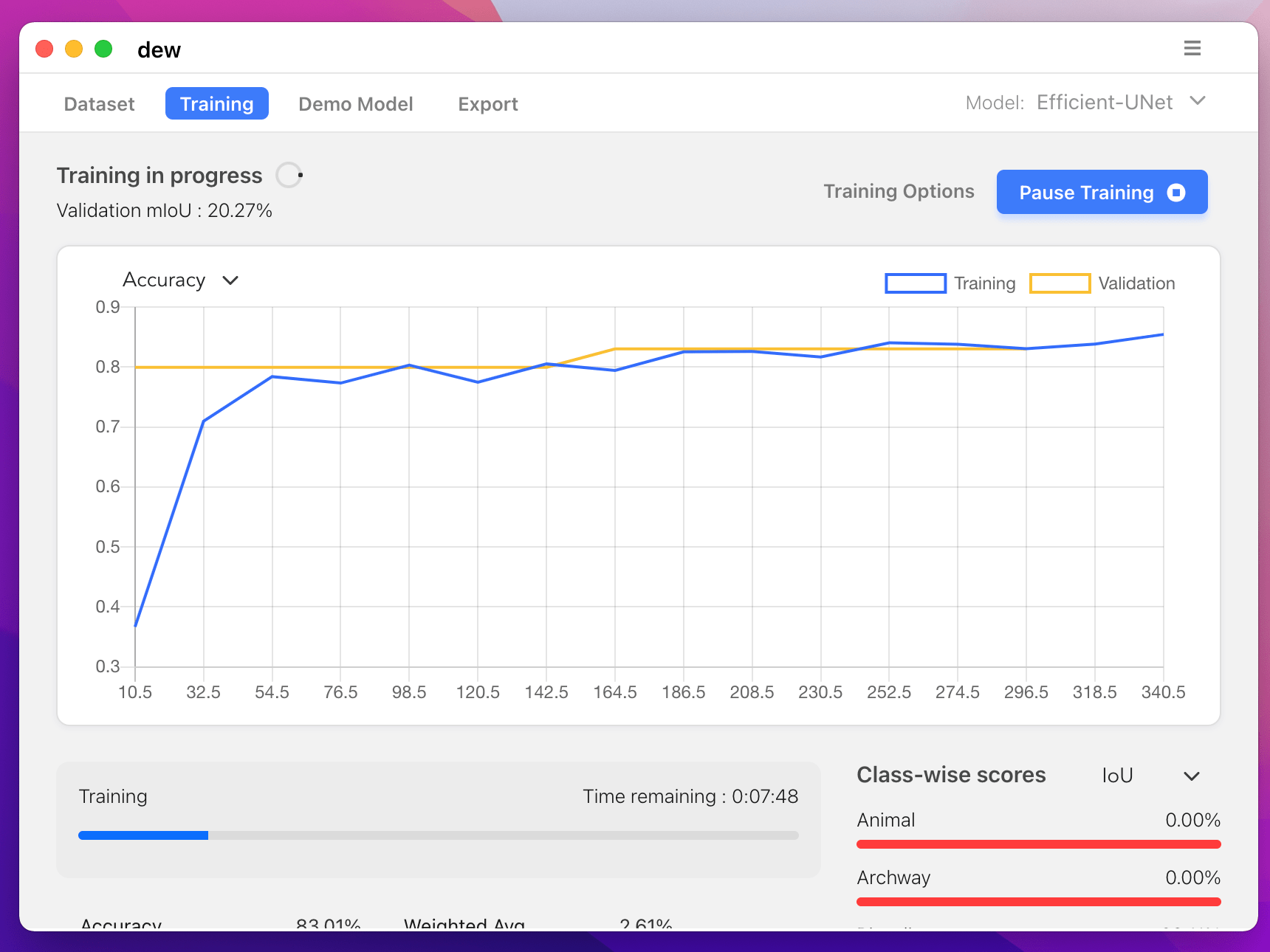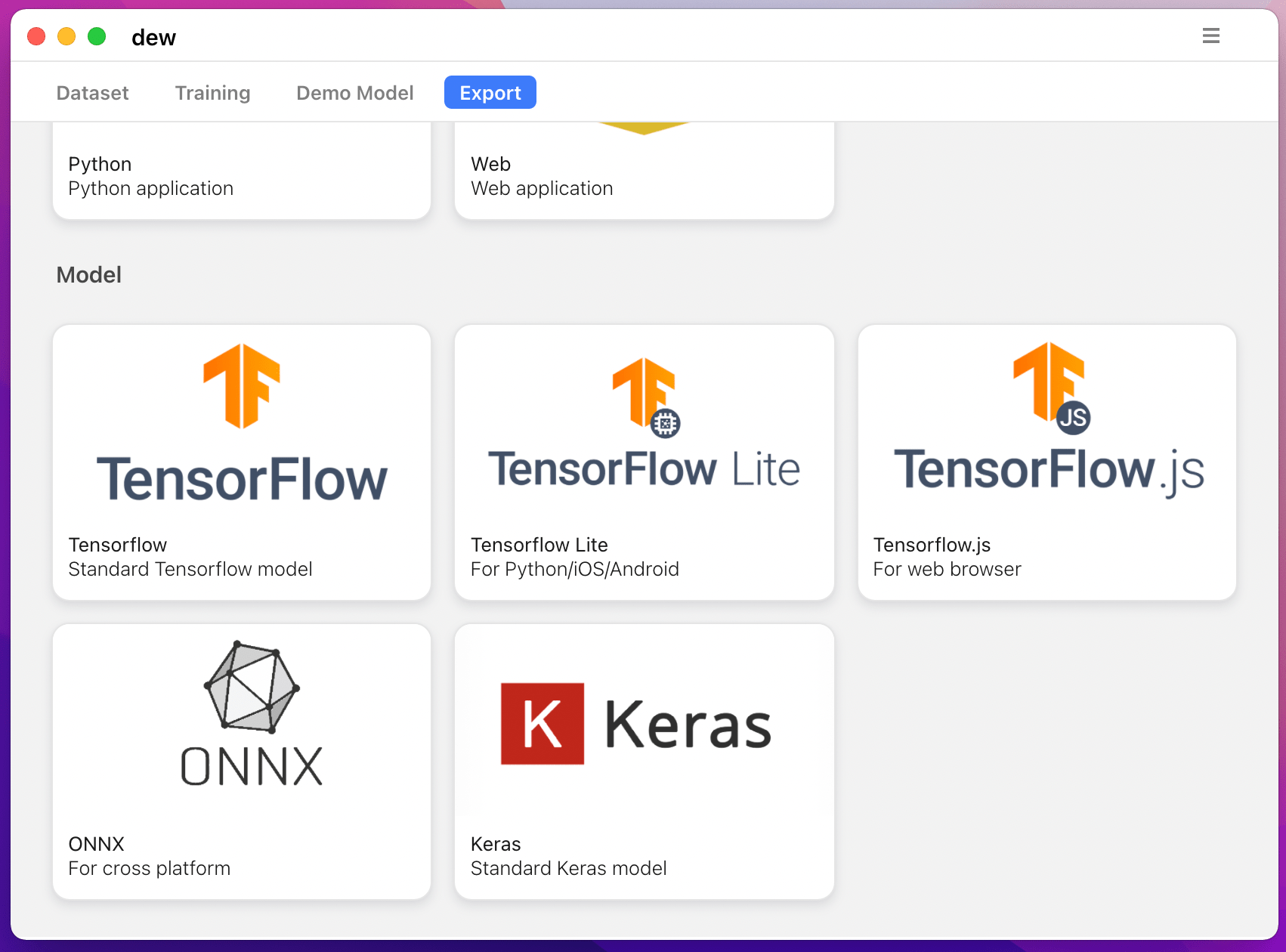## Models

Following models are supported:

model_name Base Model Segmentation Model
fcn_8 Vanilla CNN FCN8
fcn_32 Vanilla CNN FCN8
fcn_8_vgg VGG 16 FCN8
fcn_32_vgg VGG 16 FCN32
fcn_8_resnet50 Resnet-50 FCN32
fcn_32_resnet50 Resnet-50 FCN32
fcn_8_mobilenet MobileNet FCN32
fcn_32_mobilenet MobileNet FCN32
pspnet Vanilla CNN PSPNet
pspnet_50 Vanilla CNN PSPNet
pspnet_101 Vanilla CNN PSPNet
vgg_pspnet VGG 16 PSPNet
resnet50_pspnet Resnet-50 PSPNet
unet_mini Vanilla Mini CNN U-Net
unet Vanilla CNN U-Net
vgg_unet VGG 16 U-Net
resnet50_unet Resnet-50 U-Net
mobilenet_unet MobileNet U-Net
segnet Vanilla CNN Segnet
vgg_segnet VGG 16 Segnet
resnet50_segnet Resnet-50 Segnet
mobilenet_segnet MobileNet Segnet

Example results for the pre-trained models provided :

Input Image Output Segmentation Image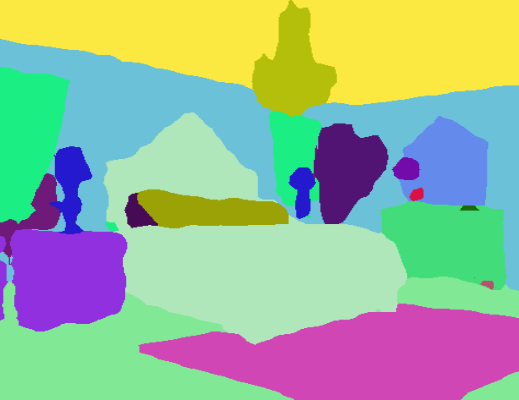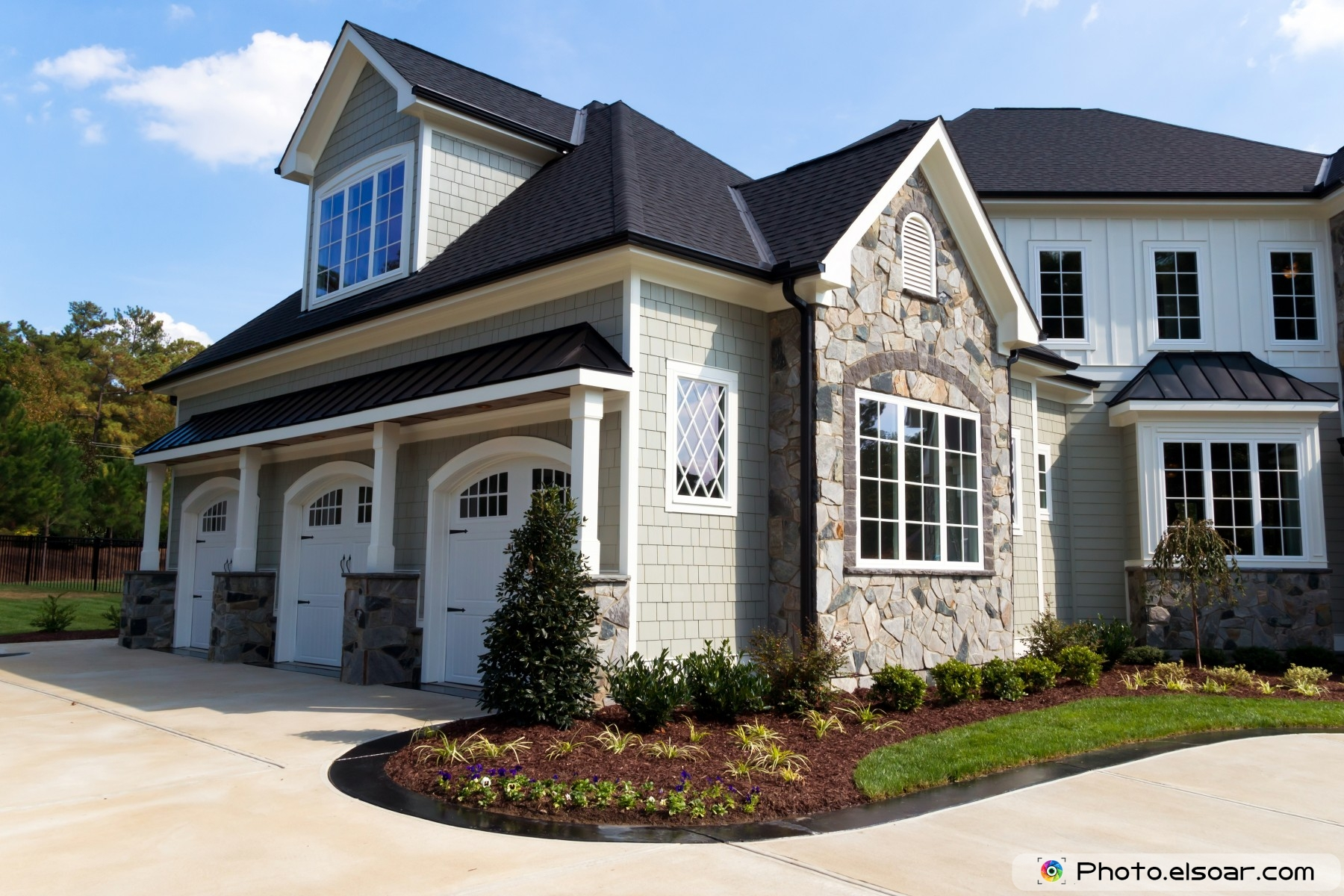## Getting Started

### Prerequisites

• Keras ( recommended version : 2.4.3 )
• OpenCV for Python
• Tensorflow ( recommended version : 2.4.1 )
``````apt-get install -y libsm6 libxext6 libxrender-dev
pip install opencv-python
``````

### Installing

Install the module

Recommended way:

``````pip install --upgrade git+https://github.com/divamgupta/image-segmentation-keras
``````

### or

``````pip install keras-segmentation
``````

### or

``````git clone https://github.com/divamgupta/image-segmentation-keras
cd image-segmentation-keras
python setup.py install
``````

## Pre-trained models:

``````from keras_segmentation.pretrained import pspnet_50_ADE_20K , pspnet_101_cityscapes, pspnet_101_voc12

model = pspnet_101_cityscapes() # load the pretrained model trained on Cityscapes dataset

model = pspnet_101_voc12() # load the pretrained model trained on Pascal VOC 2012 dataset

# load any of the 3 pretrained models

out = model.predict_segmentation(
inp="input_image.jpg",
out_fname="out.png"
)

``````

### Preparing the data for training

You need to make two folders

• Images Folder - For all the training images
• Annotations Folder - For the corresponding ground truth segmentation images

The filenames of the annotation images should be same as the filenames of the RGB images.

The size of the annotation image for the corresponding RGB image should be same.

For each pixel in the RGB image, the class label of that pixel in the annotation image would be the value of the blue pixel.

Example code to generate annotation images :

``````import cv2
import numpy as np

ann_img = np.zeros((30,30,3)).astype('uint8')
ann_img[ 3 , 4 ] = 1 # this would set the label of pixel 3,4 as 1

cv2.imwrite( "ann_1.png" ,ann_img )
``````

Only use bmp or png format for the annotation images.

You will get a folder named dataset1/

## Using the python module

You can import keras_segmentation in your python script and use the API

``````from keras_segmentation.models.unet import vgg_unet

model = vgg_unet(n_classes=51 ,  input_height=416, input_width=608  )

model.train(
train_images =  "dataset1/images_prepped_train/",
train_annotations = "dataset1/annotations_prepped_train/",
checkpoints_path = "/tmp/vgg_unet_1" , epochs=5
)

out = model.predict_segmentation(
inp="dataset1/images_prepped_test/0016E5_07965.png",
out_fname="/tmp/out.png"
)

import matplotlib.pyplot as plt
plt.imshow(out)

# evaluating the model
print(model.evaluate_segmentation( inp_images_dir="dataset1/images_prepped_test/"  , annotations_dir="dataset1/annotations_prepped_test/" ) )

``````

## Usage via command line

You can also use the tool just using command line

### Visualizing the prepared data

You can also visualize your prepared annotations for verification of the prepared data.

``````python -m keras_segmentation verify_dataset \
--images_path="dataset1/images_prepped_train/" \
--segs_path="dataset1/annotations_prepped_train/"  \
--n_classes=50
``````
``````python -m keras_segmentation visualize_dataset \
--images_path="dataset1/images_prepped_train/" \
--segs_path="dataset1/annotations_prepped_train/"  \
--n_classes=50
``````

### Training the Model

To train the model run the following command:

``````python -m keras_segmentation train \
--checkpoints_path="path_to_checkpoints" \
--train_images="dataset1/images_prepped_train/" \
--train_annotations="dataset1/annotations_prepped_train/" \
--val_images="dataset1/images_prepped_test/" \
--val_annotations="dataset1/annotations_prepped_test/" \
--n_classes=50 \
--input_height=320 \
--input_width=640 \
--model_name="vgg_unet"
``````

Choose model_name from the table above

### Getting the predictions

To get the predictions of a trained model

``````python -m keras_segmentation predict \
--checkpoints_path="path_to_checkpoints" \
--input_path="dataset1/images_prepped_test/" \
--output_path="path_to_predictions"

``````

### Video inference

To get predictions of a video

``````python -m keras_segmentation predict_video \
--checkpoints_path="path_to_checkpoints" \
--input="path_to_video" \
--output_file="path_for_save_inferenced_video" \
--display
``````

If you want to make predictions on your webcam, don't use `--input`, or pass your device number: `--input 0`
`--display` opens a window with the predicted video. Remove this argument when using a headless system.

### Model Evaluation

To get the IoU scores

``````python -m keras_segmentation evaluate_model \
--checkpoints_path="path_to_checkpoints" \
--images_path="dataset1/images_prepped_test/" \
--segs_path="dataset1/annotations_prepped_test/"
``````

## Fine-tuning from existing segmentation model

The following example shows how to fine-tune a model with 10 classes .

``````from keras_segmentation.models.model_utils import transfer_weights
from keras_segmentation.models.pspnet import pspnet_50

new_model = pspnet_50( n_classes=51 )

transfer_weights( pretrained_model , new_model  ) # transfer weights from pre-trained model to your model

new_model.train(
train_images =  "dataset1/images_prepped_train/",
train_annotations = "dataset1/annotations_prepped_train/",
checkpoints_path = "/tmp/vgg_unet_1" , epochs=5
)

``````

## Knowledge distillation for compressing the model

The following example shows transfer the knowledge from a larger ( and more accurate ) model to a smaller model. In most cases the smaller model trained via knowledge distilation is more accurate compared to the same model trained using vanilla supervised learning.

``````from keras_segmentation.predict import model_from_checkpoint_path
from keras_segmentation.models.unet import unet_mini
from keras_segmentation.model_compression import perform_distilation

model_large = model_from_checkpoint_path( "/checkpoints/path/of/trained/model" )
model_small = unet_mini( n_classes=51, input_height=300, input_width=400  )

perform_distilation ( data_path="/path/to/large_image_set/" , checkpoints_path="path/to/save/checkpoints" ,
teacher_model=model_large ,  student_model=model_small  , distilation_loss='kl' , feats_distilation_loss='pa' )

``````

## Adding custom augmentation function to training

The following example shows how to define a custom augmentation function for training.

``````
from keras_segmentation.models.unet import vgg_unet
from imgaug import augmenters as iaa

def custom_augmentation():
return  iaa.Sequential(
[
# apply the following augmenters to most images
iaa.Fliplr(0.5),  # horizontally flip 50% of all images
iaa.Flipud(0.5), # horizontally flip 50% of all images
])

model = vgg_unet(n_classes=51 ,  input_height=416, input_width=608)

model.train(
train_images =  "dataset1/images_prepped_train/",
train_annotations = "dataset1/annotations_prepped_train/",
checkpoints_path = "/tmp/vgg_unet_1" , epochs=5,
do_augment=True, # enable augmentation
custom_augmentation=custom_augmentation # sets the augmention function to use
)
``````

## Custom number of input channels

The following example shows how to set the number of input channels.

``````
from keras_segmentation.models.unet import vgg_unet

model = vgg_unet(n_classes=51 ,  input_height=416, input_width=608,
channels=1 # Sets the number of input channels
)

model.train(
train_images =  "dataset1/images_prepped_train/",
train_annotations = "dataset1/annotations_prepped_train/",
checkpoints_path = "/tmp/vgg_unet_1" , epochs=5,
# cv2.IMREAD_UNCHANGED = -1 (4 channels like RGBA)
)
``````

## Custom preprocessing

The following example shows how to set a custom image preprocessing function.

``````
from keras_segmentation.models.unet import vgg_unet

def image_preprocessing(image):
return image + 1

model = vgg_unet(n_classes=51 ,  input_height=416, input_width=608)

model.train(
train_images =  "dataset1/images_prepped_train/",
train_annotations = "dataset1/annotations_prepped_train/",
checkpoints_path = "/tmp/vgg_unet_1" , epochs=5,
preprocessing=image_preprocessing # Sets the preprocessing function
)
``````

## Custom callbacks

The following example shows how to set custom callbacks for the model training.

``````
from keras_segmentation.models.unet import vgg_unet
from keras.callbacks import ModelCheckpoint, EarlyStopping

model = vgg_unet(n_classes=51 ,  input_height=416, input_width=608 )

# When using custom callbacks, the default checkpoint saver is removed
callbacks = [
ModelCheckpoint(
filepath="checkpoints/" + model.name + ".{epoch:05d}",
save_weights_only=True,
verbose=True
),
EarlyStopping()
]

model.train(
train_images =  "dataset1/images_prepped_train/",
train_annotations = "dataset1/annotations_prepped_train/",
checkpoints_path = "/tmp/vgg_unet_1" , epochs=5,
callbacks=callbacks
)
``````

## Multi input image input

The following example shows how to add additional image inputs for models.

``````
from keras_segmentation.models.unet import vgg_unet

model = vgg_unet(n_classes=51 ,  input_height=416, input_width=608)

model.train(
train_images =  "dataset1/images_prepped_train/",
train_annotations = "dataset1/annotations_prepped_train/",
checkpoints_path = "/tmp/vgg_unet_1" , epochs=5,
other_inputs_paths=[
"/path/to/other/directory"
],

preprocessing=[lambda x: x+1, lambda x: x+2, lambda x: x+3], # Different prepocessing for each input
#     OR
preprocessing=lambda x: x+1, # Same preprocessing for each input
)
``````

## Projects using keras-segmentation

Here are a few projects which are using our library :

If you use our code in a publicly available project, please add the link here ( by posting an issue or creating a PR )

Get A Weekly Email With Trending Projects For These Topics
No Spam. Unsubscribe easily at any time.
Python (888,878
Keras (10,606
Segmentation (8,382
Resnet (3,233
Vgg (2,463
Unet (1,288
Mobilenet (1,161
Fcn (661
Segnet (245